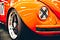# Object-Oriented JavaScript — Arrays and ConditionalsPhoto by Jen Theodore on Unsplash

JavaScript is partly an object-oriented language.

To learn JavaScript, we got to learn the object-oriented parts of JavaScript.

In this article, we’ll look at arrays and conditionals.

# Arrays

An array is a sequence of values.

It’s an object type.

It can have anything in them, including primitive values and objects.

We can define an empty array with:

`let a = [];`

And we can put values in them by writing:

`let a = [1, 2, 3];`

Array index is the position of the item. Arrays start with 0 as its index.

So the first item has index 0.

We can access any element using square brackets:

`a;`

then we get 1.

# Adding/Updating Array Elements

We can add a value to an array by assigning a value to it.

For instance, we can write:

`a = 'foo';`

Then we get:

`[1, 2, 'foo'];`

We can write:

`a = 'bar';`

Then we get:

`[1, 2, 'foo', 'bar'];`

We can have gaps in an array.

Gaps are filled with `undefined` .

Assignment can also be used to update an element.

# Arrays of Arrays

We can have an array of arrays.

For instance, we can write:

`let a = [[1, 2, 3], [4, 5, 6]];`

Then we can access entry by writing:

`a;`

We return the first entry of th first array in `a` ,. so we get 1.

We can also use the square brackets to get a character from a string, so we can write:

`let s = 'foo';`

And we get:

`s;`

which is `'f'` .

# Conditions and Loops

Conditional statements include the `if` and `switch` statements.

They let us run code depending on the condition given.

Loops include the `while` , `do...while` , `for` , `for...in` , and `for...of` loops.

# Code Blocks

A code block is a part of a piece of code that’s separated from the outside.

For instance, we can write:

`{  let a = 1;  let b = 3;}`

to create a block.

`let` lets us create variables that are only available within the block.

Blocks can be nested, so we can write:

`{  let a = 1;  let b = 3; {    let c = a + b; {      let d = a - b;    }  }}`

We have blocks that are nested in other blocks.

# if condition

We can use the `if` block to run something given a condition.

To do that, we can write:

`if (a > 3) {  result = 'a is greater than 3';}`

then the body is only run when `a` is bigger than 3.

We can have any logical expression between the parentheses.

# else Clause

The `else` clause can be added to an `if` condition if we need to run something if the `if` condition is `false` .

For example, we can write:

`if (a > 3) {  result = 'a is greater than 3';} else {  result = 'a is not greater than 3';}`

They can be nested like any other blocks.

The `if` condition is handy for checking if a variable exists.

For instance, we can write:

`if (typeof foo !== "undefined") {  result = "yes";}`

Then we check if `foo` is initialized by checking if it’s `undefined` .Photo by Markus Spiske on Unsplash

# Conclusion

Arrays are a sequence of values.

The `if` statement lets us run code conditionally.

Web developer. Subscribe to my email list now at https://thewebdev.info/subscribe/. Email me at hohanga@gmail.com

Web developer. Subscribe to my email list now at https://thewebdev.info/subscribe/. Email me at hohanga@gmail.com